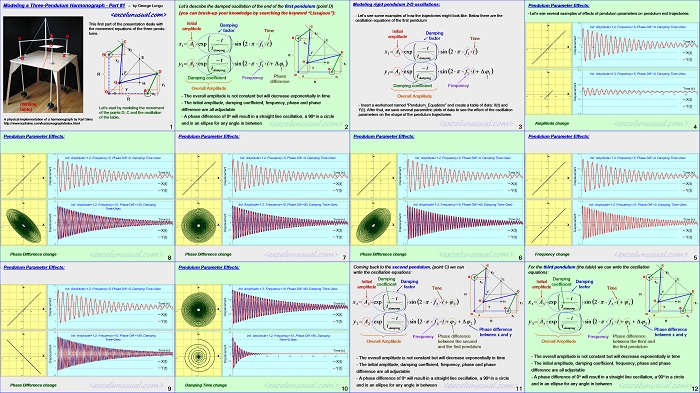# Modeling a Three-Pendulum Harmonograph – tutorial: part #1

Hi guys, here is the first part of a tutorial describing the matematical equations used in modeling a three-pendulum harmonograph (automatic drawing machine). It pertains to the second version of the model. This section refers only to the kinematic equations for the movement of three pendulums. A second section will be published later and it will describe the kinematic equations involved in the articulated linkage mechanism on the top of the table, the custom spreadsheet functions used in the model and some overall considerations about the partition of the spreadsheet and the calculations going on in the workbook and VBA. This is an advanced tutorial so you need a minimum of high-school physics and geometry background.  Please tell us your views. Cheers, George[sociallocker][/sociallocker]